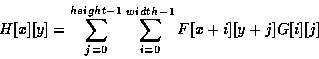Next: 13.3.2.2 Separable Filters Up: 13.3.2 The Convolution Operation Previous: 13.3.2 The Convolution Operation

#### 13.3.2.1 Two-Dimensional Convolutions

Since you generally operate on two-dimensional images in image processing, extend Equation14 to:(15)

During convolution, the value for a pixel in the output image is calculated by aligning the filter array (kernel) with the pixel at the same location in the input image and summing the values of the pixels in the input array multiplied by the corresponding values in the filter array.

The algorithm can be visualized as a loop over the width and height of the input image. In the loop, the filter is typically centered over each input pixel. Another loop over the width and height of the filter multiplies the values in the filter array with the values under the filter in the input image. The results of the multiplication are added together and stored in the output image in the same <x,y>location as the pixel in the input image. The output and input images are kept logically separate so that the results of one step in the loop don't affect later steps in the loop.

The convolution filter may have a single element per-pixel, where the RGBA components are scaled by the same value, or the filter may have separate red, green, blue, and alpha values for each element.Next: 13.3.2.2 Separable Filters Up: 13.3.2 The Convolution Operation Previous: 13.3.2 The Convolution Operation
David Blythe
1999-08-06